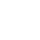CurrentHome>Resources
Occupied Bandwidth Measurement of S3302 Handheld Spectrum Analyzer
Release time :2021.03.14 Reading quantity:69 source:Salukirf, original

Saluki S3302 series handheld spectrum analyzer has the advantages of broad operating band, high performance indicators, high sweeping speed, multiple test functions, easy operation, etc. From the perspective of performance indicators, this instrument has low average noise level, low phase noise and high scanning speed.

S3302 series has a wide range of measurement functions. In this section, we mainly describe bow to use tthe occupied bandwidth measurement function of S3302 handheld spectrum analyzer to measure the occupied bandwidth of the signal.

1. Definition of occupied bandwidth

The occupied bandwidth refer to the bandwidth including energy of certain proportion to the total transmitted power, with the center frequency of the specified channel as the center. By using the occupied bandwidth measurement function of S3302 series spectrum analyzer, measurement results can be given rapidly, clearly and accurately. Depending on the modulation mode, two methods can be applied to calculate the occupied bandwidth.

(a) Power percentage: The occupied bandwidth of the signal is obtained by calculating the bandwidth of the frequency of certain percentage to the total power of the transmitted power. The power percentage can be set by the user.

(b) Power drop XdB: The occupied bandwidth in this calculation method is defined as follows: spacing between two frequency points corresponding to signal power drop by XdB on both sides of the frequency point where the signal peak power is. The signal power drop XdB can be set by the user.

2. Measurement procedures

The occupied bandwidth can be measured with S3302 series spectrum analyzer according to the following procedures:

(a) Set the signal generator to output the FM signal:

Use the signal generator to generate one FM signal. Set the frequency as 1GHz, power as -10dBm, FM offset as 500kHz and demodulation rate as 10kHz. Connect the output of the signal generator to the RF input end of the spectrum analyzer through one cable, as shown in Fig. 1. Enable the ON state of the modulation output and radio frequency.Fig.1 Schematic Diagram of Connection of Signal Generator and Spectrum Analyzer

(b) Reset the spectrum analyzer into the default state: Press [Reset].

(c) Set the center frequency: Press [Measure], [Channel Power] and [Center Freq] to set the center frequency with number keys. Set the center frequency of the spectrum analyzer as the frequency of the tested signal, i.e. 1GHz.

(d) Set the resolution bandwidth: Press [BW] and [RBW Auto Man], and set the resolution bandwidth as an appropriate value.

(e) Set the video bandwidth: Press [BW] and [VBW Auto Man], and set the video bandwidth as an appropriate value.

In order to improve the measurement accuracy, it is recommended to set the ratio of the resolution bandwidth to video bandwidth to be more than 10. Press [RBW/VBW] to change this ratio.

(f) Enable the occupied bandwidth measurement mode of the spectrum analyzer: Press [Measure], [OBW] and [OBW Off On].

After the occupied bandwidth measurement function is enabled, the spectrum analyzer will change into the occupied bandwidth measurement interface, and measurement results will be displayed at the bottom of the screen. Two vertical white lines on the screen intuitively indicates the frequency range of the occupied bandwidth. After the occupied bandwidth measurement function is enabled, the “Auto” mode of the detector will automatically change into the “Sample” mode. The user can change the measurement method, occupied bandwidth span, etc. with corresponding menus, so as to obtain more accurate measurement results.

(g) Select the measurement method:

Press [Measure], [OBW] and [Method % XdB], and select the occupied bandwidth measurement method. You can set the method as the power percentage or power drop XdB. The underline indicates the current mode, and the default setting is the percentage.

(h) Change the percentage: If the percentage method is selected, you can press [Measure], [OBW] and [% 99%], and use the number keys, UP/DOWN keys or knob to change the percentage. The percentage range is 10% to 99.99%, with the minimum step of 0.01%. The default setting is 99%.

(i) Change the XdB value: If the XdB method is selected, you can press [Measure], [OBW] and [XdB -3.00dB] and use the number keys, UP/DOWN keys or knob to change the XdB value. The XdB range is -0.1dB to -100dB, with the minimum step of 0.01dB. The default setting is -3dB.

(j) Change the occupied bandwidth span: Press [Measure], [OBW] and [Span], and enter the occupied bandwidth span with number keys. Press corresponding soft keys to enter the unit. The default setting is 3MHz.

(k) Disable the occupied bandwidth measurement: Press [Measure], [OBW] and [OBW Off On], and disable the occupied bandwidth measurement. The interface will change into the spectrum measurement interface.